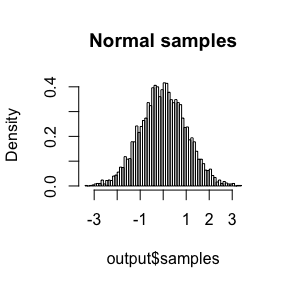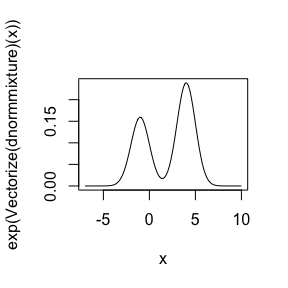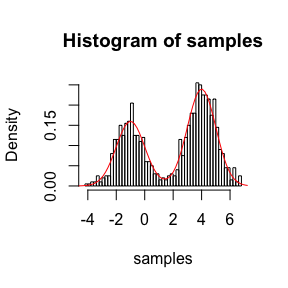# Background

Adaptive Rejection Metropolis Sampling (ARMS) is a Markov chain Monte Carlo based algorithm to sample from a univariate target distribution specified by its (potentially unnormalised) log density. The algorithm constructs a rejection distribution based on piecewise linear functions that envelop the log density of the target. For distributions with log concave density functions, this envelope is used directly, and usually results in a very efficient sampler. For distributions that are not log concave, or have unknown log concavity, an extra Metropolis Hastings accept/reject step is used to correct for any mismatch between the proposal density and the target. This sometimes results in a sampler with good convergence properties.

This R package provides an efficient C++ reimplementation of ARMS.

# Using the R package

You can run ARMS by calling the arms function. Usage is best illustrated by examples, given in the next two sections.

## Example: normal distribution

A very simple example, for which exact sampling is possible, is the unit normal distribution. This has the unnormalised log density of $$-\frac{x^2}{2}$$, with the entire real line as its domain, and the density is log concave. This means we can use metropolis = FALSE to get exact independent samples:

output <- arms(
5000, function(x) -x ^ 2 / 2,
-1000, 1000,
metropolis = FALSE, include_n_evaluations = TRUE
)
print(str(output))
#> List of 2
#>  $n_evaluations: int 197 #>$ samples      : num [1:5000] -0.0269 -0.3525 -0.4471 -1.6393 -0.3032 ...
#> NULL
hist(output$samples, br = 50, freq = FALSE, main = 'Normal samples')shapiro.test(output$samples)
#>
#>  Shapiro-Wilk normality test
#>
#> data:  output\$samples
#> W = 0.99969, p-value = 0.6795

(Note: there are obviously better ways to sample this distribution—rnorm for a start.)

## Example: mixture of normal distributions

Another simple example, but one for which the Metropolis-Hastings step is required, is a mixture of normal distributions. For instance, consider

$x \sim 0.4 N(-1, 1) + 0.6 N(4, 1),$

which has a density that looks like

dnormmixture <- function(x) {
parts <- log(c(0.4, 0.6)) + dnorm(x, mean = c(-1, 4), log = TRUE)
log(sum(exp(parts - max(parts)))) + max(parts)
}

curve(exp(Vectorize(dnormmixture)(x)), -7, 10)This distribution is not log-concave, so we need to use metropolis = TRUE to correct the inexactness caused by the use of an imperfect rejection distribution. Doing this we can sample from the distribution

samples <- arms(1000, dnormmixture, -1000, 1000)
hist(samples, freq = FALSE, br = 50)
curve(exp(Vectorize(dnormmixture)(x)), -7, 10, col = 'red', add = TRUE)# Using the C++ implementation

The R package contains a header-only C++ implementation of the algorithm, which can be used in other packages. To use it, add this package (armspp) to the LinkingTo for your package, then #include <armspp> in a C++ file. You can find an example for how to call this function in the src/armspp.cpp of this package.